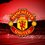# A beautiful sum!

$\sum_{n=1}^{\infty} \left(\frac\psi(n)-\psi\left(n-\frac{1}{2}\right)}\binom{4n-2}{2n-1}}+\frac\psi\left(n+\frac{1}{2}\right)-\psi(n)}\binom{4n}{2n}}\right) = \frac{\pi}{3\sqrt{3}}+\frac{1}{75}\left(\log(1073741824)-3\sqrt{5} \pi^2+\pi\left(\Re \{{4 i \sqrt{5} \log(5- \sqrt{5} + i (\sqrt{15}- \sqrt{3}) ) - 2 i \sqrt{5} \log(5 +\sqrt{5}+ i (\sqrt{3} + \sqrt{15}))\}}+6\sqrt{5}\arctan\left(\sqrt{\frac{5}{3}}\right)-5\sqrt{3}\right)+\sqrt{5}\left(\log(79228162514264337593543950336) \log\left(\frac{3-\sqrt{5}}{2}\right) + \log(324518553658426726783156020576256)\log\left(\frac{3+\sqrt{5}}{2}\right)+24\Re \left\{\operatorname{Li}_2\left(\frac{\sqrt{3}+i}{\sqrt{3}+i\sqrt{5}}\right)-\operatorname{Li}_2\left(\frac{\sqrt{3}+i}{\sqrt{3}-i\sqrt{5}}\right)\right\}\right)\right$

Prove the above sum.

I found this sum unanswered in another mathematics forum. Can this be done without computational aid? Any ideas of solving this would be accepted.3 years, 7 months ago

This discussion board is a place to discuss our Daily Challenges and the math and science related to those challenges. Explanations are more than just a solution — they should explain the steps and thinking strategies that you used to obtain the solution. Comments should further the discussion of math and science.

When posting on Brilliant:

• Use the emojis to react to an explanation, whether you're congratulating a job well done , or just really confused .
• Ask specific questions about the challenge or the steps in somebody's explanation. Well-posed questions can add a lot to the discussion, but posting "I don't understand!" doesn't help anyone.
• Try to contribute something new to the discussion, whether it is an extension, generalization or other idea related to the challenge.

MarkdownAppears as
*italics* or _italics_ italics
**bold** or __bold__ bold
- bulleted- list
• bulleted
• list
1. numbered2. list
1. numbered
2. list
Note: you must add a full line of space before and after lists for them to show up correctly
paragraph 1paragraph 2

paragraph 1

paragraph 2

[example link](https://brilliant.org)example link
> This is a quote
This is a quote
    # I indented these lines
# 4 spaces, and now they show
# up as a code block.

print "hello world"
# I indented these lines
# 4 spaces, and now they show
# up as a code block.

print "hello world"
MathAppears as
Remember to wrap math in $$ ... $$ or $ ... $ to ensure proper formatting.
2 \times 3 $2 \times 3$
2^{34} $2^{34}$
a_{i-1} $a_{i-1}$
\frac{2}{3} $\frac{2}{3}$
\sqrt{2} $\sqrt{2}$
\sum_{i=1}^3 $\sum_{i=1}^3$
\sin \theta $\sin \theta$
\boxed{123} $\boxed{123}$

Sort by:

It is known that $\psi(n) = H_{n-1} - \gamma$ for all $n \in \mathbb{N}$. With a little work, we can also show that $\psi\left(n+\frac{1}{2}\right) = 2H_{2n} - H_n - \gamma - \ln(4)$.

Therefore, we can rewrite the sum as: $\sum_{n=1}^{\infty} \left( \dfrac{2H_{n-1} - 2H_{2n-2} + \gamma + \ln(4) }{\binom{4n-2}{2n-1}} + \dfrac{2H_{2n} - 2H_n + \frac{1}{n} - \gamma - \ln(4)}{\binom{4n}{2n}} \right)$

We can break these down into a few different sums, all of which obviously converge:

$= \sum_{n=1}^{\infty} \dfrac{\gamma + \ln(4)}{\binom{4n-2}{2n-1}} - \sum_{n=1}^{\infty} \dfrac{\gamma + \ln(4)}{\binom{4n}{2n}} - \sum_{n=1}^{\infty} \dfrac{2H_{n-1} }{\binom{4n-2}{2n-1} } + \sum_{n=1}^{\infty} \dfrac{2H_{2n-2} }{ \binom{4n-2}{2n-1}} + \sum_{n=1}^{\infty} \dfrac{2H_{2n} }{\binom{4n}{2n} } - \sum_{n=1}^{\infty} \dfrac{2H_n}{\binom{4n}{2n} } + \sum_{n=1}^{\infty} \dfrac{1}{n \binom{4n}{2n}}$

We can calculate the first two sums using the following McLauren Series:

$f(x) = \sum_{k=1}^{\infty} \dfrac{(-1)^{k+1} x^{2k}}{\binom{2k}{k}} = \dfrac{4x \text{arcsinh}\left(\dfrac{x}{2}\right)}{(4+x^2)^{3/2}} + \dfrac{x^2}{4+x^2}$

Then, $\sum_{n=1}^{\infty} \dfrac{\gamma + \ln(4)}{\binom{4n-2}{2n-1}} - \sum_{n=1}^{\infty} \dfrac{\gamma + \ln(4)}{\binom{4n}{2n}} = (\gamma + \ln(4))f(1)$

Of course that's just the beginning of the calculations, and it's already pretty complicated. I'm not sure how to calculate the sum of a harmonic number over a binomial coefficient.

- 3 years, 6 months ago